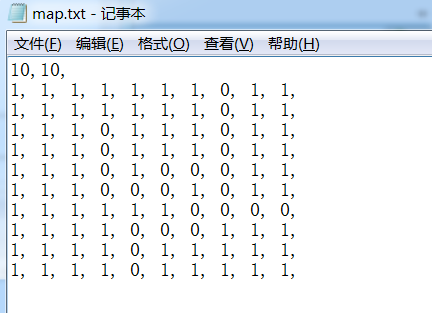# C++利用循环和栈实现走迷宫

更新时间：2020年03月19日 15:22:06   作者：春风来不来

1、将地图的数组保存在文件中，从文件中读取行列数

2.、动态开辟空间保存地图

3.、运行结束后再地图上标出具体的走法

1、文件中第一行分别放置的是地图的行数和列数

2、其中1表示墙，即路不通，0表示路，即通路

3、程序运行结束后用2标记走过的路径

4、当走到“死胡同”时用3标记此路为死路

5、每到一个点，按照 左 上 右 下 的顺序去试探

6、没有处理入口就是"死胡同"的极端情况```#ifndef _MAZE_H_
#define _MAZE_H_

#include <stack>
#include <fstream> // ifstream
#include <iostream>
#include <string>
using namespace std;

// 坐标类
class Seat
{
public:
Seat(int _x, int _y)
:x(_x)
,y(_y)
{ }
int x;
int y;
};

// 迷宫类

class Maze
{
private:
int** _map; // 指向地图的指针
int _row; // 存放地图的行数
int _col; // 存放地图的列数
public:
// 构造函数 读取文件里的地图 和行数
Maze(const string& filePath);

private:
// 判断是否是路
bool IsPass(Seat& entry);

public:
// 开始走
bool PassMaze(stack<Seat>& s, Seat& entry);

// 打印地图
void PrintMap();

// 析构 释放空间
~Maze();
};
#endif
```

maze.cpp

```// 迷宫之递归实现
#include "maze.h"

// 构造函数
Maze::Maze(const string& filePath)
{
ifstream mapFile(filePath, ofstream::out);
assert(mapFile); // 断言文件是否存在
string str_row_col; //第一行 获取行和列
string str_temp; // 临时字符串

// 获取行列的个数
getline(mapFile,str_row_col);// 读取第一行
str_temp = str_row_col.substr(0, str_row_col.find_first_of(','));// 取得字符串中的字串获取行数
_row = atoi(str_temp.c_str()); // atoi将字符串转为数字
str_temp = str_row_col.substr(str_row_col.find_first_of(',')+1); // 取得字符串中的列数
_col = atoi(str_temp.c_str()); // atoi将字符串转为数字

// 分配空间
_map = new int*[_row];
for (int idx = 0; idx < _col; ++idx)
{
_map[idx] = new int[_col];
}

// 填充地图
int index_row = 0; // 放置地图 二维数组的行索引
int index_col = 0; // 放置地图 二维数组的列索引
while (!mapFile.eof())
{
getline(mapFile, str_temp);
char* a_line = (char*)str_temp.c_str();
// 遍历一行
while (*a_line != '\0')
{
if (*a_line == '0' || *a_line == '1')
{
_map[index_row][index_col++] = *a_line - '0'; // 减0 是将字符'0'的 ASCII对应的十进制值减去
}
a_line++; // 向后移动指针
}
++index_row;
index_col = 0; // 每处理完一行后将列索引置0
}
mapFile.close();
}

// 判断是否是路
bool Maze::IsPass(Seat& entry)
{
if (entry.x < 0 || entry.y < 0 || entry.y >= _col || entry.x >= _row)
{
return true;
}
if (_map[entry.x][entry.y] == 0)
{
return true;
}
return false;
}

// 开始走
void Maze::PassMaze( Seat& entry)
{
stack<Seat> s;
if (IsPass(entry))
{
s.push(entry); // 压栈当前位置
while (!s.empty()) // 栈不为空继续
{
Seat curSeat = s.top(); // 取得栈顶存储的位置
// 走到边界
if (curSeat.x < 0 || curSeat.y < 0 || entry.y >= _col || entry.x >= _row )
{
return;
}
_map[curSeat.x][curSeat.y] = 2; // 将走过的路标记为2

// 往左走
Seat left(curSeat.x, curSeat.y-1);
if (IsPass(left))
{
s.push(left);
continue;
}
// 往上走
Seat up(curSeat.x-1, curSeat.y);
if (IsPass(up))
{
s.push(up);
continue;
}
// 往右走
Seat right(curSeat.x, curSeat.y+1);
if (IsPass(right))
{
s.push(right);
continue;
}
// 往下走
Seat down(curSeat.x+1, curSeat.y);
if (IsPass(down))
{
s.push(down);
continue;
}

// 运行到此处说明 每个方向都没有路可走 是死路标记为3
_map[curSeat.x][curSeat.y] = 3;
// 出栈这个“死路位置”
s.pop();
}
}
}

// 打印地图
void Maze::PrintMap()
{
for (int index_row = 0; index_row < _row; ++index_row)
{
for (int index_col = 0; index_col < _col; ++index_col)
{
cout << _map[index_row][index_col] <<" ";
}
cout <<endl;
}
cout <<endl;
}

// 析构
Maze::~Maze()
{
for (int idx = 0; idx < _row; ++idx )
{
delete[] _map[idx];
}
delete[] _map;
_map = NULL;
}

test.cpp
[cpp] view plain copy
int main()
{
Maze m1("map.txt"); // 构造一个迷宫对象
m1.PrintMap(); // 走之前打印
m1.PassMaze(Seat(9, 4)); //开始走 传递迷宫入口点
m1.PrintMap(); // 结束后再次打印
return 0;
}

```